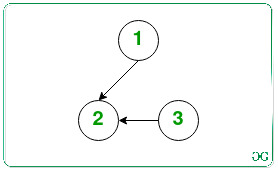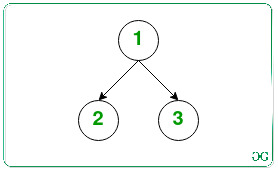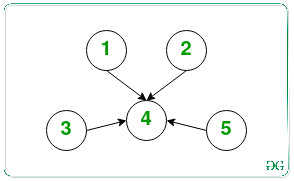Related Articles
Check if every node can me made accessible from a node of a Tree by at most N/2 given operations
• Last Updated : 13 Apr, 2021

Given a Directed Tree consisting of N nodes, the task is to check if there exists a node in the given tree such that all other nodes are reachable by removing any directed edge from the Tree and adding another directed edge between any pair of nodes in the Tree at most floor(N/2) times. If there exists any such node, then print “Yes”. Otherwise, print “No”.

Examples:

Input: N = 3Output: Yes
Explanation:
Remove the edge 2 -> 3 and insert an edge 1 -> 3.Therefore, both the remaining nodes (2, 3) are now accessible from the node 1.
Count of operations required is 1, which is <= floor(3/2) (= 1).
Input: N = 5Output: No

Approach: The idea to solve this problem is based on the following observations:

• Every node should have at least one parent node, i.e. every node should have at least 1 indegree to make the tree accessible from the required node.
• It can be concluded that if every node has at least 1 indegree, then all other nodes can be accessed.
• Therefore, the task reduces to finding the number of nodes having 0 in-degrees and check if it is at most N / 2 or not.

Follow the steps below to solve the problem:

Below is the implementation of the above approach:

## C++

 `// C++ program for the above approach``#include``using` `namespace` `std;` `void` `findNode(map<``int``, ``int``> mp, ``int` `n)``{``    ` `    ``// Store the indegree``    ``// of every node``    ``int` `a[n];` `    ``for``(``int` `i = 0; i < n; i++)``    ``{``        ``a[i] = mp[i + 1];``    ``}` `    ``// Store the nodes having``    ``// indegree equal to 0``    ``int` `count0 = 0;` `    ``// Traverse the array``    ``for``(``int` `i = 0; i < n; i++)``    ``{``        ` `        ``// If the indegree``        ``// of i-th node is 0``        ``if` `(a[i] == 0)``        ``{``            ` `            ``// Increment count0 by 1``            ``count0++;``        ``}``    ``}` `    ``count0 -= 1;` `    ``// If the number of operations``    ``// needed is at most floor(n/2)``    ``if` `(count0 <= ``floor``(((``double``)n) /``                        ``((``double``)2)))``    ``{``        ``cout << ``"Yes"``;``    ``}` `    ``// Otherwise``    ``else``        ``cout << ``"No"``;``}` `// Driver Code``int` `main()``{``    ` `    ``// Given number of nodes``    ``int` `N = 3;` `    ``// Given Directed Tree``    ``map<``int``, ``int``> mp;``    ``mp = 0;``    ``mp = 2;``    ``mp = 0;` `    ``findNode(mp, N);``}` `// This code is contributed by SURENDRA_GANGWAR`

## Java

 `// Java program for the above approach``import` `java.io.*;``import` `java.util.HashMap;` `class` `GFG {` `    ``// Function to check if there is a``    ``// node in tree from where all other``    ``// nodes are accessible or not``    ``public` `static` `void``    ``findNode(HashMap map,``             ``int` `n)``    ``{` `        ``// Store the indegree``        ``// of every node``        ``int``[] a = ``new` `int``[n];` `        ``for` `(``int` `i = ``0``; i < n; i++) {``            ``a[i] = map.getOrDefault(i + ``1``, ``0``);``        ``}` `        ``// Store the nodes having``        ``// indegree equal to 0``        ``int` `count0 = ``0``;` `        ``// Traverse the array``        ``for` `(``int` `i = ``0``; i < n; i++) {` `            ``// If the indegree``            ``// of i-th node is 0``            ``if` `(a[i] == ``0``) {` `                ``// Increment count0 by 1``                ``count0++;``            ``}``        ``}` `        ``count0 -= ``1``;` `        ``// If the number of operations``        ``// needed is at most floor(n/2)``        ``if` `(count0``            ``<= Math.floor(((``double``)n)``                          ``/ ((``double``)``2``))) {``            ``System.out.println(``"Yes"``);``        ``}` `        ``// Otherwise``        ``else``            ``System.out.println(``"No "``);``    ``}` `    ``// Driver Code``    ``public` `static` `void` `main(String[] args)``    ``{``        ``// Given number of nodes``        ``int` `N = ``3``;` `        ``// Given Directed Tree``        ``HashMap map``            ``= ``new` `HashMap<>();` `        ``map.put(``1``, ``0``);``        ``map.put(``2``, ``2``);``        ``map.put(``3``, ``0``);` `        ``findNode(map, N);``    ``}``}`
Output:
`Yes`

Time Complexity: O(N)
Auxiliary Space: O(N)

Attention reader! Don’t stop learning now. Get hold of all the important DSA concepts with the DSA Self Paced Course at a student-friendly price and become industry ready.

My Personal Notes arrow_drop_up